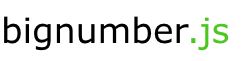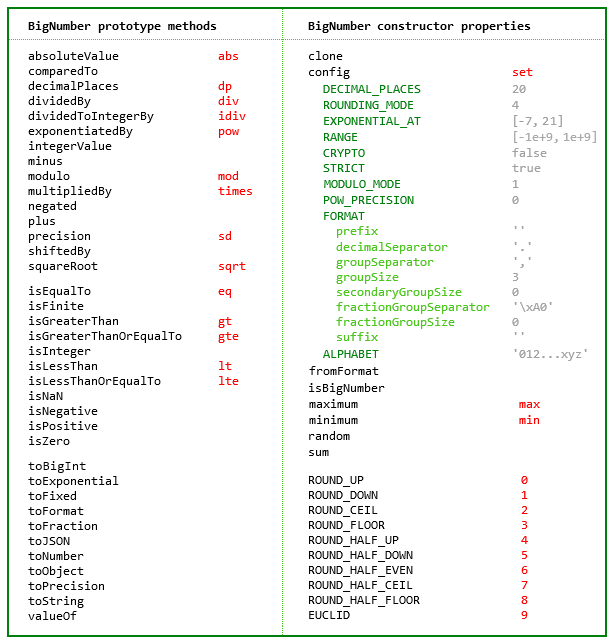# MikeMcl/bignumber.js

A JavaScript library for arbitrary-precision decimal and non-decimal arithmetic
Latest commit 2601c3e Feb 24, 2019
Type Name Latest commit message Commit time
Failed to load latest commit information.doc Feb 23, 2019perf Oct 31, 2018test Feb 23, 2019.npmignore Jan 8, 2017.travis.yml Nov 1, 2018CHANGELOG.md Feb 24, 2019LICENCE Jan 13, 2019README.md Feb 23, 2019bignumber.d.ts Feb 24, 2019bignumber.js Feb 24, 2019bignumber.min.js Feb 24, 2019bignumber.min.js.map Feb 23, 2019bignumber.mjspackage.json Feb 24, 2019A JavaScript library for arbitrary-precision decimal and non-decimal arithmetic.## Features

• Integers and decimals
• Simple API but full-featured
• Faster, smaller, and perhaps easier to use than JavaScript versions of Java's BigDecimal
• 8 KB minified and gzipped
• Replicates the `toExponential`, `toFixed`, `toPrecision` and `toString` methods of JavaScript's Number type
• Includes a `toFraction` and a correctly-rounded `squareRoot` method
• Supports cryptographically-secure pseudo-random number generation
• No dependencies
• Wide platform compatibility: uses JavaScript 1.5 (ECMAScript 3) features only
• Comprehensive documentation and test setIf a smaller and simpler library is required see big.js. It's less than half the size but only works with decimal numbers and only has half the methods. It also does not allow `NaN` or `Infinity`, or have the configuration options of this library.

See also decimal.js, which among other things adds support for non-integer powers, and performs all operations to a specified number of significant digits.

The library is the single JavaScript file bignumber.js (or minified, bignumber.min.js).

Browser:

`<script src='path/to/bignumber.js'></script>`
`\$ npm install bignumber.js`
`const BigNumber = require('bignumber.js');`

ES6 module:

`import BigNumber from "./bignumber.mjs"`

AMD loader libraries such as requireJS:

```require(['bignumber'], function(BigNumber) {
// Use BigNumber here in local scope. No global BigNumber.
});```

## Use

The library exports a single constructor function, `BigNumber`, which accepts a value of type Number, String or BigNumber,

```let x = new BigNumber(123.4567);
let y = BigNumber('123456.7e-3');
let z = new BigNumber(x);
x.isEqualTo(y) && y.isEqualTo(z) && x.isEqualTo(z);      // true```

To get the string value of a BigNumber use `toString()` or `toFixed()`. Using `toFixed()` prevents exponential notation being returned, no matter how large or small the value.

```let x = new BigNumber('1111222233334444555566');
x.toString();                       // "1.111222233334444555566e+21"
x.toFixed();                        // "1111222233334444555566"```

If the limited precision of Number values is not well understood, it is recommended to create BigNumbers from String values rather than Number values to avoid a potential loss of precision.

In all further examples below, `let`, semicolons and `toString` calls are not shown. If a commented-out value is in quotes it means `toString` has been called on the preceding expression.

```// Precision loss from using numeric literals with more than 15 significant digits.
new BigNumber(1.0000000000000001)         // '1'
new BigNumber(88259496234518.57)          // '88259496234518.56'
new BigNumber(99999999999999999999)       // '100000000000000000000'

// Precision loss from using numeric literals outside the range of Number values.
new BigNumber(2e+308)                     // 'Infinity'
new BigNumber(1e-324)                     // '0'

// Precision loss from the unexpected result of arithmetic with Number values.
new BigNumber(0.7 + 0.1)                  // '0.7999999999999999'```

When creating a BigNumber from a Number, note that a BigNumber is created from a Number's decimal `toString()` value not from its underlying binary value. If the latter is required, then pass the Number's `toString(2)` value and specify base 2.

`new BigNumber(Number.MAX_VALUE.toString(2), 2)`

BigNumbers can be created from values in bases from 2 to 36. See `ALPHABET` to extend this range.

```a = new BigNumber(1011, 2)          // "11"
b = new BigNumber('zz.9', 36)       // "1295.25"
c = a.plus(b)                       // "1306.25"```

Performance is better if base 10 is NOT specified for decimal values. Only specify base 10 when it is desired that the number of decimal places of the input value be limited to the current `DECIMAL_PLACES` setting.

A BigNumber is immutable in the sense that it is not changed by its methods.

```0.3 - 0.1                           // 0.19999999999999998
x = new BigNumber(0.3)
x.minus(0.1)                        // "0.2"
x                                   // "0.3"```

The methods that return a BigNumber can be chained.

```x.dividedBy(y).plus(z).times(9)
x.times('1.23456780123456789e+9').plus(9876.5432321).dividedBy('4444562598.111772').integerValue()```

Some of the longer method names have a shorter alias.

```x.squareRoot().dividedBy(y).exponentiatedBy(3).isEqualTo(x.sqrt().div(y).pow(3))    // true
x.modulo(y).multipliedBy(z).eq(x.mod(y).times(z))                                   // true```

As with JavaScript's Number type, there are `toExponential`, `toFixed` and `toPrecision` methods.

```x = new BigNumber(255.5)
x.toExponential(5)                  // "2.55500e+2"
x.toFixed(5)                        // "255.50000"
x.toPrecision(5)                    // "255.50"
x.toNumber()                        //  255.5```

A base can be specified for `toString`. Performance is better if base 10 is NOT specified, i.e. use `toString()` not `toString(10)`. Only specify base 10 when it is desired that the number of decimal places be limited to the current `DECIMAL_PLACES` setting.

`x.toString(16)                     // "ff.8"`

There is a `toFormat` method which may be useful for internationalisation.

```y = new BigNumber('1234567.898765')
y.toFormat(2)                       // "1,234,567.90"```

The maximum number of decimal places of the result of an operation involving division (i.e. a division, square root, base conversion or negative power operation) is set using the `set` or `config` method of the `BigNumber` constructor.

The other arithmetic operations always give the exact result.

```BigNumber.set({ DECIMAL_PLACES: 10, ROUNDING_MODE: 4 })

x = new BigNumber(2)
y = new BigNumber(3)
z = x.dividedBy(y)                        // "0.6666666667"
z.squareRoot()                            // "0.8164965809"
z.exponentiatedBy(-3)                     // "3.3749999995"
z.toString(2)                             // "0.1010101011"
z.multipliedBy(z)                         // "0.44444444448888888889"
z.multipliedBy(z).decimalPlaces(10)       // "0.4444444445"```

There is a `toFraction` method with an optional maximum denominator argument

```y = new BigNumber(355)
pi = y.dividedBy(113)               // "3.1415929204"
pi.toFraction()                     // [ "7853982301", "2500000000" ]
pi.toFraction(1000)                 // [ "355", "113" ]```

and `isNaN` and `isFinite` methods, as `NaN` and `Infinity` are valid `BigNumber` values.

```x = new BigNumber(NaN)                                           // "NaN"
y = new BigNumber(Infinity)                                      // "Infinity"
x.isNaN() && !y.isNaN() && !x.isFinite() && !y.isFinite()        // true```

The value of a BigNumber is stored in a decimal floating point format in terms of a coefficient, exponent and sign.

```x = new BigNumber(-123.456);
x.c                                 // [ 123, 45600000000000 ]  coefficient (i.e. significand)
x.e                                 // 2                        exponent
x.s                                 // -1                       sign```

For advanced usage, multiple BigNumber constructors can be created, each with their own independent configuration.

```// Set DECIMAL_PLACES for the original BigNumber constructor
BigNumber.set({ DECIMAL_PLACES: 10 })

// Create another BigNumber constructor, optionally passing in a configuration object
BN = BigNumber.clone({ DECIMAL_PLACES: 5 })

x = new BigNumber(1)
y = new BN(1)

x.div(3)                            // '0.3333333333'
y.div(3)                            // '0.33333'```

For further information see the API reference in the doc directory.

## Test

The test/modules directory contains the test scripts for each method.

The tests can be run with Node.js or a browser. For Node.js use

``````\$ npm test
``````

or

``````\$ node test/test
``````

To test a single method, use, for example

``````\$ node test/methods/toFraction
``````

For the browser, open test/test.html.

## Build

For Node, if uglify-js is installed

``````npm install uglify-js -g
``````

then

``````npm run build
``````

will create bignumber.min.js.

A source map will also be created in the root directory.

## Feedback

Open an issue, or email

Michael

## Licence

The MIT Licence.

See LICENCE.

You can’t perform that action at this time.